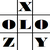anything in Excel...

It's Excel-o-lozy...

### Nearest to Average - How to get a value closest to the average value of a data series?

Suppose there is a data series.
An average value is calculated from the series.
Now a value, Nearest / Closest to the average value, is to be taken out from the data series.

Think HOW?
Simple…

Just paste the following formula in any cell:

=IF(ABS(SMALL(D3:D6,COUNT(D3:D6)/2)-AVERAGE(D3:D6))>LARGE(D3:D6,COUNT(D3:D6)/2)-AVERAGE(D3:D6),LARGE(D3:D6,COUNT(D3:D6)/2),SMALL(D3:D6,COUNT(D3:D6)/2))

here "D3:D6" is the range of data.

Simply complex... or
Compellingly Simple...

See the example below (Copy this table to Excel)
 A B C D E F G 1 2 Explanation Data Formula 3 4 Data series 2 5 3 6 6 7 8 8 Total 19 9 10 Average 4.75 =AVERAGE(B3:B6) 11 12 Closest to average 6.00 =IF(ABS(SMALL(B3:B6,COUNT(B3:B6)/2)-AVERAGE(B3:B6))>LARGE(B3:B6,COUNT(B3:B6)/2)-AVERAGE(B3:B6),LARGE(B3:B6,COUNT(B3:B6)/2),SMALL(B3:B6,COUNT(B3:B6)/2))

a Screen shot...

Liked it???